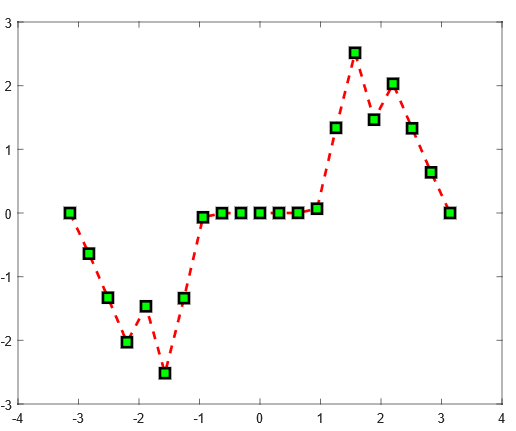Main Content

# Prevent MATLAB Figure Display During Report Generation

This example shows how to prevent the display of MATLAB® figures in MATLAB during report generation. If you generate a report that includes several MATLAB figures, you can avoid the overhead of displaying the figures as you create them.

The example creates and includes these MATLAB figures in a report. When the figures are created in MATLAB, the display of the figures is suppressed.Import the Report API package so that you do not have to use long, fully-qualified class names.

```import mlreportgen.report.* ```

Create a Word report. You can run this example with other report types by changing the output type.

• To create a single-file HTML report, change the output type to `'html-file'`.

• To create a multi-file HTML report, change the output type to `'html'`.

• To create a PDF report, change the output type to `'pdf'`.

```rpt = Report('InvisibleFigure','docx'); ```

Add a title page and table of contents to the report.

```add(rpt,TitlePage('Title','Display Invisible Figures','Author','John Doe')); add(rpt,TableOfContents); ```

Create a chapter and add a figure to it. To prevent the display of the figure in MATLAB, set the `Visible` property of the figure to `'off'`.

```ch = Chapter('Invisible Figure 1'); x = -pi:pi/10:pi; y = tan(sin(x)) - sin(tan(x)); f1 = figure('visible','off'); plot(x,y,'--rs','LineWidth',2,... 'MarkerEdgeColor','k',... 'MarkerFaceColor','g',... 'MarkerSize',10) add(ch,Figure(f1)); add(rpt,ch); ```

Create a second chapter and add an invisible figure to it.

```ch = Chapter('Invisible Figure 2'); f2 = figure('visible','off'); surf(peaks); add(ch,Figure(f2)); add(rpt,ch); ```

Close and view the report.

```close(rpt); rptview(rpt); ```

## SupportGet trial now